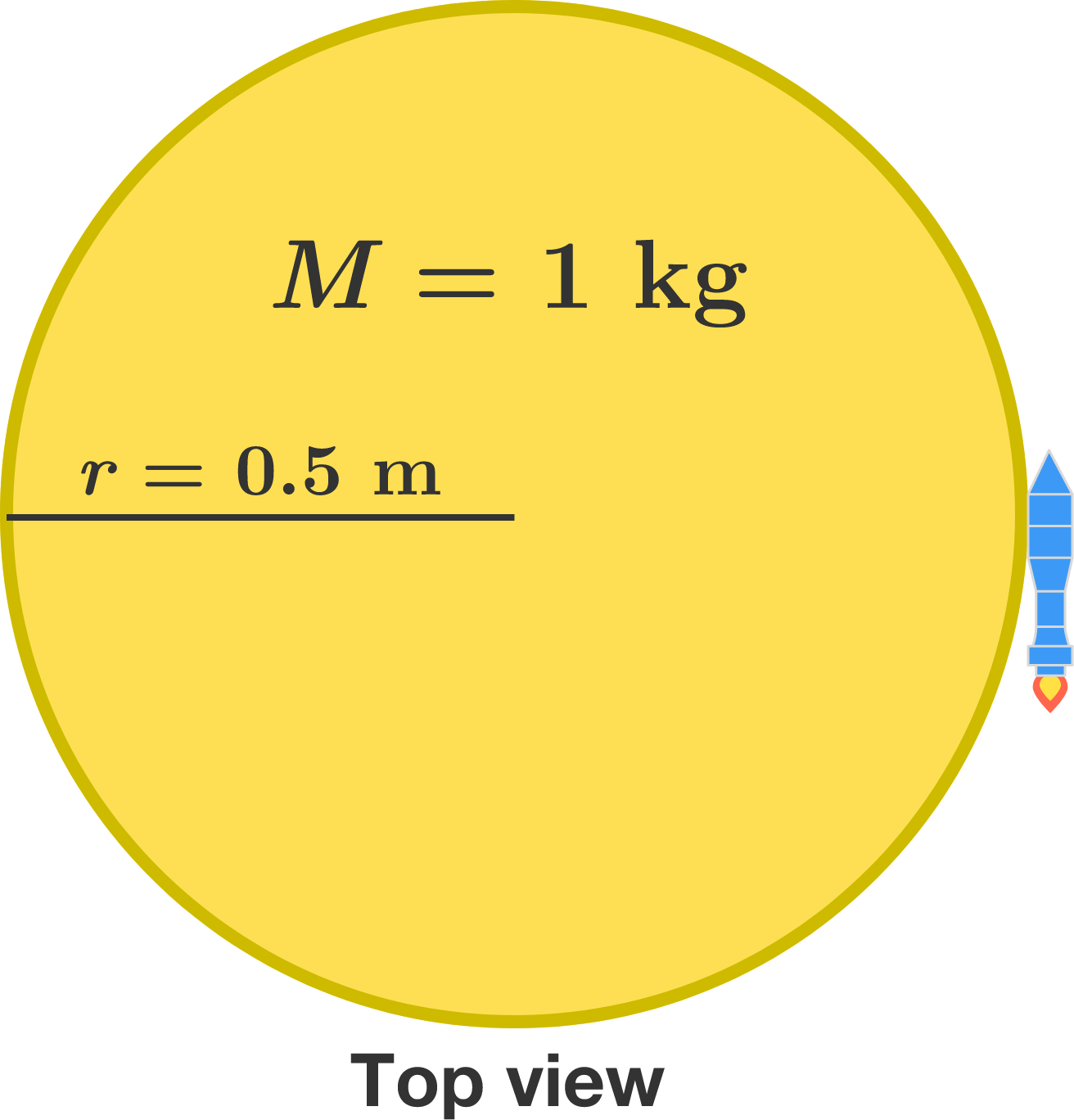# Unbalanced ThrustA uniform disk sits on a smooth floor with a rocket strapped to its perimeter. One second after the rocket ignites, how far is the disk's center from where it started, in meters?


Details and Assumptions:

• The mass of the disk is $M=\SI{1}{\kilo\gram},$ its radius is $r=\SI{0.5}{\meter},$ and the rocket provides a constant thrust of $T = \SI{10}{\newton}$ after it ignites.
• Neglect the mass and size of the rocket.